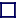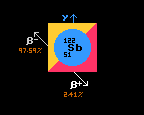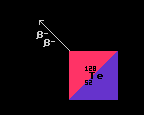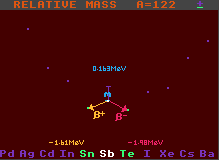Dual mode decay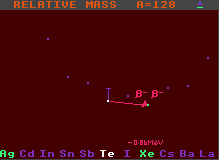Double beta decay

### EVEN MASS BETA STABILITY

EVEN ATOMIC WEIGHT ISOBARS and DUAL-MODE DECAYS or DOUBLE BETA STABILITY
The atomic masses of isobars having an even atomic weight lie alternately on one of two nested parabolae, one of higher atomic mass than the other. Such an arrangement produces two mass minima, with two isobars having minimum mass, allowing either dual mode decay, or double beta decay.

In dual-mode decay, the isobar lying between the two isobars with minimum mass can decay by either of two routes, beta decay or inverse beta decay, the probability of each being measured as the branching ratio or branching factor.

In double beta decay, the next isobar has a higher energy, but the second removed has a lower energy, and the selected nuclide is able to decay (very slowly) if two beta decays occur simultaneously. This is a highly improbable occurrence, so the halflives are extremely long. It cannot decay by just one beta decay, as it would then end up having more mass, not less. 36 possible candidates for double beta decay can be found in the chart, but the decays are so rare that many have never been observed to decay and are officially classified as being stable.RELATIVE MASS of A=122 ISOBARS
Fig 1 shows a cross section of isobars of atomic weight 122, with antimony-122 having higher mass the the two neighbouring isobars on either side, tin-122 and tellurium-122. The antimony thus has a choice of either undergoing beta decay to become tellurium-122 releasing 1.98MeV of energy or of undergoing inverse beta decay and becoming tin-122 releasing 1.61MeV of energy.RELATIVE MASS of A=128 ISOBARS
Fig 2 shows a cross section of isobars of atomic weight 128, with tellurium-128 decaying by double beta decay into the stable xenon-128 releasing 860KeV of energy and two electrons. Note that it cannot decay into iodine-128 because that has more mass than tellurium-128. The halflife of Te-128 is 5×1024years.